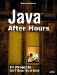Making the Fish Swim

 The swim method is what gets those fish to go. It does this by incrementing the location x and y coordinates by adding the x and y values stored in the velocity variable each time swim is called. Every so often, the fish should change direction, just as fish in an aquarium might. Here's how that works in the swim method, which, every now and then, adds new random numbers to the x and y component of the velocity and then makes sure no velocity component exceeds an absolute magnitude of 8: public void swim() { if(random.nextInt() % 7 <= 1){ velocity.x += random.nextInt() % 4; velocity.x = Math.min(velocity.x, 8); velocity.x = Math.max(velocity.x, -8); velocity.y += random.nextInt() % 4; velocity.y = Math.min(velocity.y, 8); velocity.y = Math.max(velocity.y, -8); } . . . Next, the code adds the x and y components of the fish's velocity to move the fish to a new position: location.x += velocity.x; location.y += velocity.y; All that's left to do is to determine if updating the fish's position has put it beyond the edge of the aquarium, in which case the fish should bounce off the edge of the tank, which you can make happen by reversing the sign of its x or y velocity, like this: if (location.x < edges.x) { location.x = edges.x; velocity.x = -velocity.x; } if ((location.x + image1.getWidth(tank)) > (edges.x + edges.width)){ location.x = edges.x + edges.width - image1.getWidth(tank); velocity.x = -velocity.x; } if (location.y < edges.y){ location.y = edges.y; velocity.y = -velocity.y; } if ((location.y + image1.getHeight(tank)) > (edges.y + edges.height)){ location.y = edges.y + edges.height - image1.getHeight(tank); velocity.y = -velocity.y; } } That completes the swim method, which updates the fish's position each time it's called. The last thing to do is to actually draw the fish when needed, and that's done by the drawFishImage method.Java After Hours: 10 Projects Youll Never Do at Work
ISBN: 0672327473
EAN: 2147483647
Year: 2006
Pages: 128

Similar book on Amazon